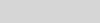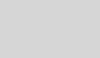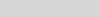# The JIT finally proposed. JIT and SIMD are getting married.Immo

Processor speed no longer follows Moore’s law. So in order to optimize the performance of your applications, it’s increasingly important to embrace parallelization. Or, as Herb Sutter phrased it, the free lunch is over.

You may think that task-based programming or offloading work to threads is already the answer. While multi-threading is certainly a critical part, it’s important to realize that it’s still important to optimize the code that runs on each core. SIMD is a technology that employs data parallelization at the CPU level. Multi-threading and SIMD complement each other: multi-threading allows parallelizing work over multiple cores while SIMD allows parallelizing work within a single core.

Today we’re happy to announce a new preview of RyuJIT that provides SIMD functionality. The SIMD APIs are exposed via a new NuGet package, Microsoft.Bcl.Simd, which is also released as a preview.

Here is an example on how you would use it:

``// Initalize some vectorsVector<float> values = GetValues();Vector<float> increment = GetIncrement();// The next line will leverage SIMD to perform the// addition of multiple elements in parallel:Vector<float> result = values + increment;``

# What’s SIMD and why do I care?

SIMD is by far the most popular code gen request and still a fairly popular request overall (~2,000 votes on user voice):It’s so popular because, for certain kinds of apps, SIMD offers a profound speed-up. For example, the performance of rendering Mandelbrot can be improved a lot by using SIMD: it improves by a factor of 2-3 (using SSE2-capable hardware) up to a factor of 4-5 (using AVX-capable hardware).# Introduction to SIMD

SIMD stands for “single instruction, multiple data”. It’s a set of processor instructions that operate over vectors instead of scalars. This allows mathematical operations to execute over a set of values in parallel.

At a high-level, SIMD enables data parallelization at the CPU level. For example, imagine you need to increment a set of floating point numbers by a given value. Normally, you’d write a `for` loop to perform this operation sequentially:

``float[] values = GetValues();float increment = GetIncrement();// Perform increment operation as manual loop:for (int i = 0; i < values.Length; i++){    values[i] += increment;}``

SIMD allows adding multiple values simultaneously by using CPU specific instructions. This is often exposed as a vector operation:

``Vector<float> values = GetValues();Vector<float> increment = GetIncrement();// Perform addition as a vector operation:Vector<float> result = values + increment;``

It’s interesting to note that there isn’t a single SIMD specification. Rather, each processor has a specific implementation of SIMD. They differ by the number of elements that can be operated on as well as by the set of available operations. The most commonly available implementation of SIMD on Intel/AMD hardware is SSE2.

Here is a simplified model of how SIMD is exposed at the CPU level:

1. There are SIMD-specific CPU registers. They have a fixed size. For SSE2, the size is 128 bit.

2. The processor has SIMD-specific instructions, specific to the operand size. As far as the processor is concerned, a SIMD value is just a bunch of bits. However, a developer wants to treat those bits as a vector of, say, 32-bit integer values. For this purpose, the processor has instructions that are specific to the operation, e.g. addition, and the operand type, e.g. 32-bit integers.

An area where SIMD operations are very useful is graphics and gaming as:

• These apps are very computation-intensive.
• Most of the data structures are already represented as vectors.

However, SIMD is applicable to any application type that performs numerical operations on a large set of values; this also includes scientific computing and finance.

# Designing SIMD for .NET

Most .NET developers don’t have to write CPU-specific code. Instead, the CLR abstracts the hardware by providing a virtual machine that translates your code into machine instructions, either at runtime (JIT) or at install time (NGEN). By leaving the code generation to the CLR, you can share the same MSIL between machines with different processors without having to give up on CPU-specific optimizations.

This separation is what enables a library ecosystem because it tremendously simplifies code sharing. We believe the library ecosystem is a key part of why .NET is such a productive environment.

In order to keep this separation, we needed to come up with a programming model for SIMD that allows you to express vector operations without tying you to a specific processor implementation, such as SSE2. We came up with a model that provides two categories of vector types:Both categories of types are what we call JIT intrinsics. That means the JIT knows about these types and treats them specially when emitting machine code. However, all types are also designed to work perfectly in cases where the hardware doesn’t support SIMD (unlikely today) or the application doesn’t use this new version of RyuJIT.

Our goal is to ensure the performance in those cases is roughly identical to sequentially written code. Unfortunately, in this preview we aren’t there yet.

## Vectors with a fixed size

Let’s talk about the fixed-size vectors first. There are many apps that already define their own vector types, especially graphic intense applications, such as games or a ray tracer. In most cases these apps use single-precision floating point values.

The key aspect is that those vectors have a specific number of elements, usually two, three or four. The two-element vectors are often used to represent points or similar entities, such as complex numbers. Vectors with three and four elements are typically used for 3D (the 4th element is used to make the math work). The bottom line is that those domains require vectors with a specific number of elements.

To get a sense how these types look, look at the simplified shape of `Vector3f`:

``public struct Vector3f{    public Vector3f(float value);    public Vector3f(float x, float y, float z);    public float X { get; }    public float Y { get; }    public float Z { get; }    public static bool operator ==(Vector3f left, Vector3f right);    public static bool operator !=(Vector3f left, Vector3f right);    // With SIMD, these element wise operations are done in parallel:    public static Vector3f operator +(Vector3f left, Vector3f right);    public static Vector3f operator -(Vector3f left, Vector3f right);    public static Vector3f operator -(Vector3f value);    public static Vector3f operator *(Vector3f left, Vector3f right);    public static Vector3f operator *(Vector3f left, float right);    public static Vector3f operator *(float left, Vector3f right);    public static Vector3f operator /(Vector3f left, Vector3f right);}``

I’d like to highlight the following aspects:

• We’ve designed the fixed size vectors so that they can easily replace the ones defined in apps.
• For performance reasons, we’ve defined those types as immutable value types.
• The idea is that after replacing your vector with our vector, your app behaves the same, except that it runs faster. For more details, have a look at our Ray Tracer sample application.

## Vectors with a hardware dependent size

While the fixed size vector types are convenient to use, their maximum degree of parallelization is limited by the number of components. For example, an application that uses `Vector2f` can get a speed-up of at most a factor of two – even if the hardware would be capable of performing operations on eight elements at a time.

In order for an application to scale with the hardware capabilities, the developer has to vectorize the algorithm. Vectorizing an algorithm means that the developer needs to break the input into a set of vectors whose size is hardware-dependent. On a machine with SSE2, this means the app could operate over vectors of four 32-bit floating point values. On a machine with AVX, the same app could operate over vectors with eight 32-bit floating point values.

To get a sense for the differences, here is a simplified version of the shape of `Vector<T>`:

``public struct Vector<T> where T : struct {    public Vector(T value);    public Vector(T[] values);    public Vector(T[] values, int index);    public static int Length { get; }    public T this[int index] { get; }    public static bool operator ==(Vector<T> left, Vector<T> right);    public static bool operator !=(Vector<T> left, Vector<T> right);     // With SIMD, these element wise operations are done in parallel:    public static Vector<T> operator +(Vector<T> left, Vector<T> right);    public static Vector<T> operator &(Vector<T> left, Vector<T> right);    public static Vector<T> operator |(Vector<T> left, Vector<T> right);    public static Vector<T> operator /(Vector<T> left, Vector<T> right);    public static Vector<T> operator ^(Vector<T> left, Vector<T> right);    public static Vector<T> operator *(Vector<T> left, Vector<T> right);    public static Vector<T> operator *(Vector<T> left, T right);    public static Vector<T> operator *(T left, Vector<T> right);    public static Vector<T> operator ~(Vector<T> value);    public static Vector<T> operator -(Vector<T> left, Vector<T> right);    public static Vector<T> operator -(Vector<T> value);}``

Key aspects of this type include the following:

• It’s generic. To increase flexibility and avoid combinatorial explosion of types, we’ve defined the hardware-dependent vector as a generic type, `Vector<T>`. For practical reasons, `T` can only be a primitive numeric type. In this preview, we only support `int`, `long`, `float` and `double`. The final version will also include support for all other integral numeric types, including their unsigned counterparts.

• The length is static. Since the length is hardware -dependent but fixed, the length is exposed via a static `Length` property. The value is defined as `sizeof(SIMD-register) / sizeof(T)`. In other words, two vectors `Vector<T1>` and `Vector<T2>` have the same length if `T1` and `T2` have the same size. This allows us to correlate elements in vectors of different element types, which is a very useful property in vectorized code.

Vectorization is a complicated topic and, as such, it’s well beyond the scope of this blog post. Nonetheless, let me give you a high-level overview of what this would mean for a specific app. Let’s look at a Mandelbrot renderer. Conceptually, Mandelbrot works over complex numbers which can be represented as vectors with two elements. Based on a mathematical algorithm, these complex numbers are color-coded and rendered as a single point in the resulting picture.

In the naïve usage of SIMD, one would vectorize the algorithm by representing the complex numbers as a `Vector2f`. A more sophisticated algorithm would vectorize over the points to render (which is unbounded) instead of the dimension (which is fixed). One way to do it is to present the real and imaginary components as vectors. In other words, one would vectorize the same component over multiple points.

For more details, have a look at our Mandelbrot sample. In particular, compare the scalar version to the vectorized version.

# Using the SIMD preview

In this preview we provide the following two pieces:

1. A new release of RyuJIT that provides SIMD support
2. A new NuGet library that exposes the SIMD support

The NuGet library was explicitly designed to work without SIMD support provided by the hardware/JIT. In that case, all methods and operations are implemented as pure IL. However, you obviously only get the best performance when using this library in conjunction with the new release of RyuJIT.

In order to use SIMD, you need to perform the following steps:

2. Set some environment variables to enable the new JIT and SIMD for your process. The easiest way to do this is by creating a batch file that starts your application:

``@echo offset COMPLUS_AltJit=*set COMPLUS_FeatureSIMD=1start myapp.exe``
3. Add a reference to the Microsoft.Bcl.Simd NuGet package. You can do this by right clicking your project and selecting Manage NuGet References. In the following dialog make sure you select the tab named Online. You also need to select Include Prelease in drop down at the top. Then use the textbox in the top right corner to search for Microsoft.Bcl.Simd. Click Install.

Since this a preview, there are certain limitations you may want to be aware of:

• SIMD is only enabled for 64-bit processes. So make sure your app either is targeting x64 directly or is compiled as Any CPU and not marked as 32-bit preferred.

• The Vector type only supports int, long, float and double. Instantiating `Vector<T>` with any other type will cause a type load exception.

• SIMD is only taking advantage of SSE2 hardware, currently. Due to some implementation restrictions, the RyuJIT CTP cannot automatically switch the size of the type based on local hardware capability. Full AVX support should arrive with a release of the .NET runtime that includes RyuJIT. We have prototyped this, but it just doesn’t work with our CTP deployment model.

# Summary

We’ve released a preview that brings the power of SIMD to the world of managed code. The programming model is exposed via a set of vector types, made available via the new Microsoft.Bcl.Simd NuGet package. The processor support for the operations is provided with the new preview of RyuJIT.

We’d love to get your feedback on both pieces. How do you like the programming model? What’s missing? How does the performance look like for your app? Please use the comments for providing feedback or send us mail at ryujit(at)microsoft.com.